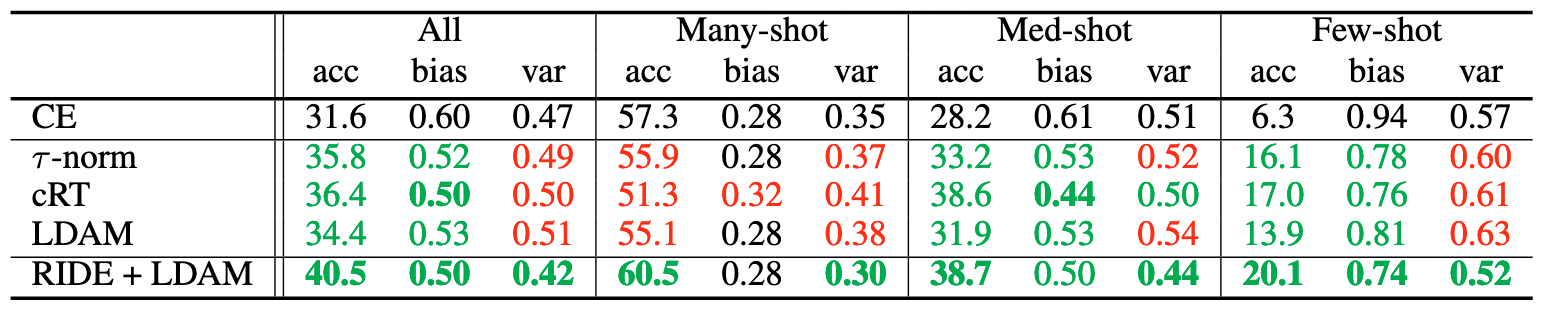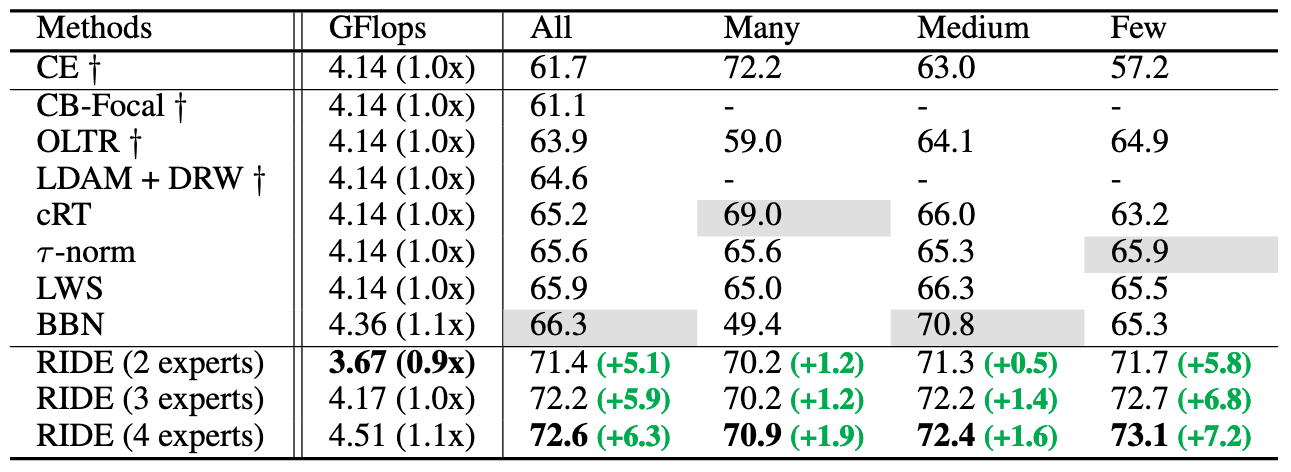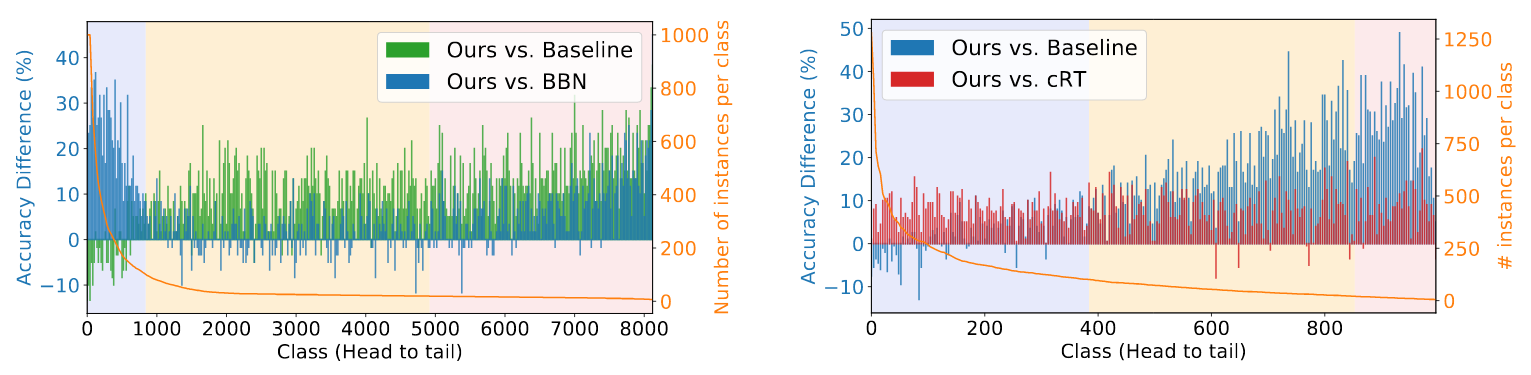# Long-tailed Recognition by Routing Diverse Distribution-Aware Experts

## ABSTRACT

-----

Natural data are often long-tail distributed over semantic classes. Existing recognition methods tend to focus on tail performance gain, often at the expense of head performance loss from increased classifier variance. The low tail performance manifests itself in large inter-class confusion and high classifier variance. We aim to reduce both the bias and the variance of a long-tailed classifier by RoutIng Diverse Experts (RIDE). It has three components: 1) a shared architecture for multiple classifiers (experts); 2) a distribution-aware diversity loss that encourages more diverse decisions for classes with fewer training instances; and 3) an expert routing module that dynamically assigns more ambiguous instances to additional experts. With on-par computational complexity, RIDE significantly outperforms the state-of-the-art methods by 5% to 7% on all the benchmarks including CIFAR100-LT, ImageNet-LT and iNaturalist. RIDE is also a universal framework that can be applied to different backbone networks and integrated into various long-tailed algorithms and training mechanisms for consistent performance gains.

To our best knowledge, RIDE is the first paper that increases the performances on all three splits (many-/med-/few-shot).

## METHOD

-----

#### Motivation

Comparedto the standard cross-entropy (CE) classifier, existing SOTA methods almost always increase the variance and some reduce the tail bias at the cost of increasing the head variance.Fig 1. The proposed method RIDE gains accuracies over SOTA by reducing both model bias and variance.

#### Method Overview

RIDE applies a two-stage optimization process. a) We first jointly optimize multiple diverse experts with distribution-aware diversity loss. b) An expert assignment module that could dynamically assign "ambiguous" samples to extra experts is trained in stage two. At test time, we combine the predictions of assigned experts to form a robust prediction. Since tail classes are inclined to be confused with other classes, by adding the expert assignment module, the data imbalance ratio for later experts can be automatically reduced without any distribution-aware loss, which allows focusing less on confident head classes and more on tail classes.Fig 2. Framework.

#### Distribution-aware Diversity Loss

The distribution-aware diversity loss is proposed to penalize the inter-expert correlation, formulated as:

$${ \mathcal{L}_{\text{D-Diversify}}^i = - \frac{\lambda}{k-1}\sum_{j\neq i}^n\mathcal{D}_{KL}(\phi^i(\vec{x},\vec{T}), \phi^j(\vec{x},\vec{T})) }$$

$$\text{where } \phi^i(\vec{x},\vec{T}) = \text{softmax}(\psi_{{\theta}_i}(f_{\theta}(\vec{x}))/\vec{T})$$, $$i$$ is the expert index, $$\mathcal{D}_{KL}(\cdot, \cdot)$$ is the KL-Divergence ($$\mathcal{D}_{KL}(P, Q) = \sum_{x \in \mathcal{X}}P(x)\log{\frac{P(x)}{Q(x)}}$$), $$\lambda$$ is the balancing factor between diversity and classification loss between ground truth label $$\vec{y}$$ and model predictions, $$\vec{T}$$ is temperatures of each sample and division is performed as element-wise division, and $$\mathcal{X}$$ is the probability space of $$P(x)$$ and $$Q(x)$$. The temperature $$T_i$$ for samples in class $$i$$ can be calculated by:

$${ {T_i} = \eta\psi_i + \eta(1-\max(\Psi)); {\Psi} = \{\psi_1, ...,\psi_C\} = \{\gamma\cdot C\cdot\frac{n_i}{\sum_{k=1}^{C} n_k} + (1-\gamma)\}_{i=1}^{C} }$$

#### Total Loss for Stage One

$${ \mathcal{L}_{\text{Total}}^i = \mathcal{L}^i_{\text{Classify}}(\phi^i(\vec{x}), y) - \frac{\lambda}{n-1}\sum_{j \neq i}^n\mathcal{D}_{KL}(\phi^i(\vec{x}, \vec{T}), \phi^j(\vec{x}, \vec{T})) }$$

where $$i$$ is the expert index, $$\mathcal{L}^i_{\text{Classify}}(.,.)$$ can be LDAM loss, focal loss, etc., depending on the training mechanisms we choose.

#### Routing Loss

The expert assignment module is optimized with the routing loss, a weighted variant of binary cross entropy loss:

$${ \mathcal{L}_{\text{Routing}} = -\omega_{\text{p}} y\log(\frac{1}{1+e^{-y_{\text{ea}}}}) - \omega_{\text{n}}(1 - y)\log(1-\frac{1}{1+e^{-y_{\text{ea}}}}) }$$

## Results

-----Fig 3. Top-1 accuracy comparison with state-of-the-arts on CIFAR100-LT with an imbalance ratio of 100.Fig 4. Extend RIDE to various long-tailed recognition methods. Consistent improvements can be observed on CIFAR100-LT.Fig 5. Top-1 accuracy comparison with state-of-the-art methods on ImageNet-LT with ResNet-50 and ResNeXt-50.Fig 6. Comparison with state-of-the-art methods on iNaturalist.Fig 7. The absolute accuracy difference of RIDE (blue) over iNaturalist's current SOTA BBN (left) and ImageNet-LT's current SOTA method cRT (right)

-----

## CITATION

-----

@article{wang2020long,
title={Long-tailed Recognition by Routing Diverse Distribution-Aware Experts},
author={Wang, Xudong and Lian, Long and Miao, Zhongqi and Liu, Ziwei and Yu, Stella X},
journal={arXiv preprint arXiv:2010.01809},
year={2020}
}